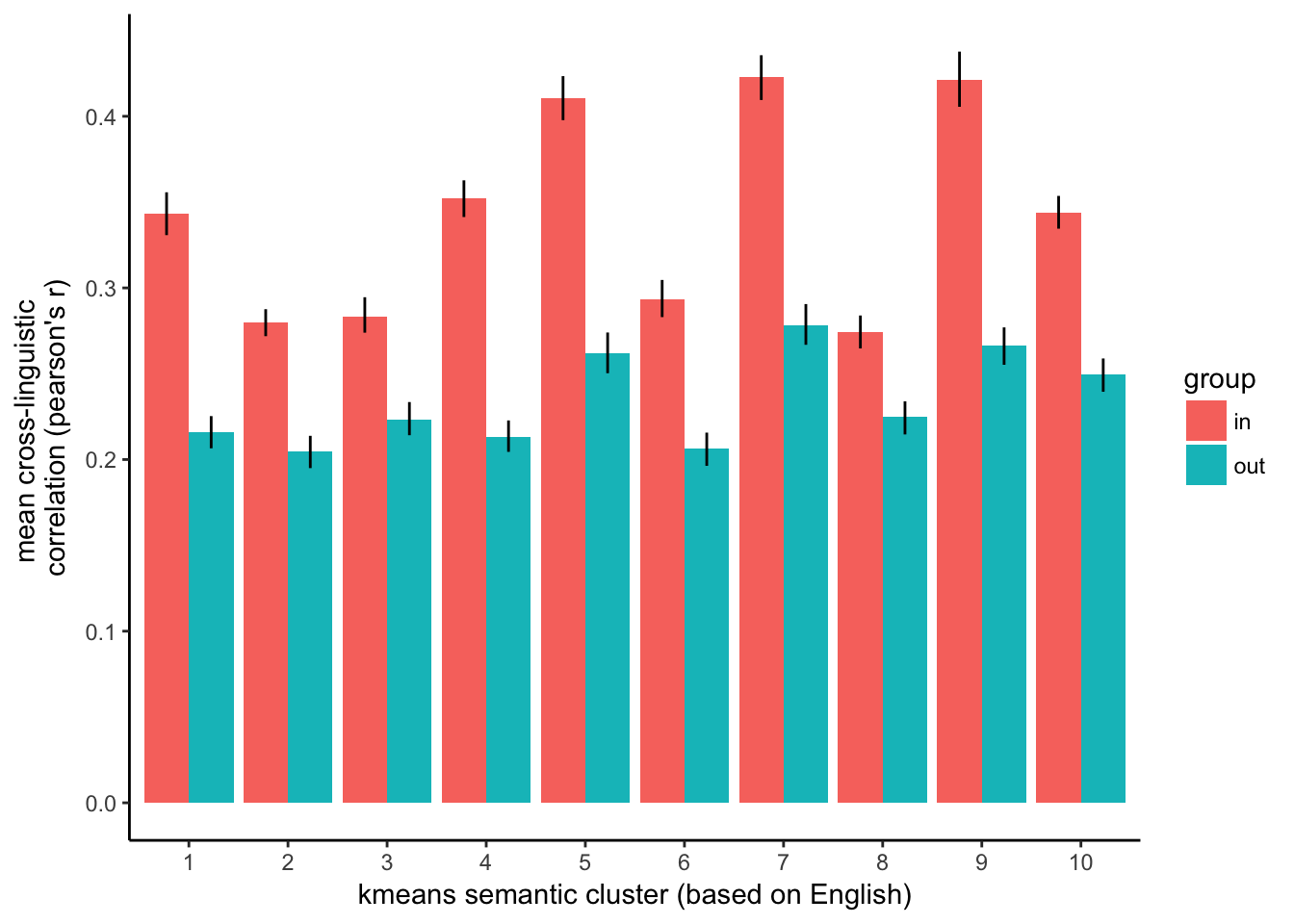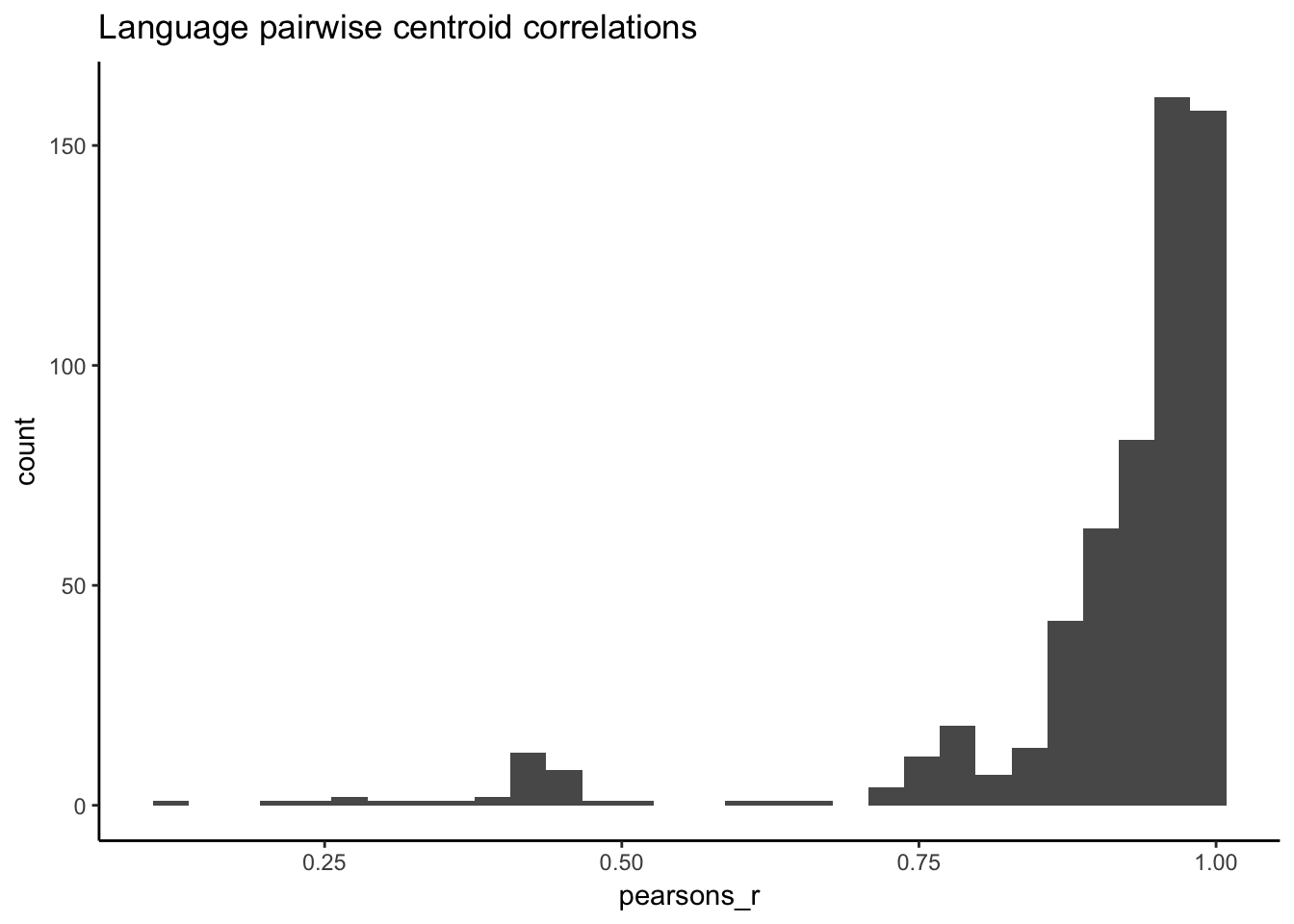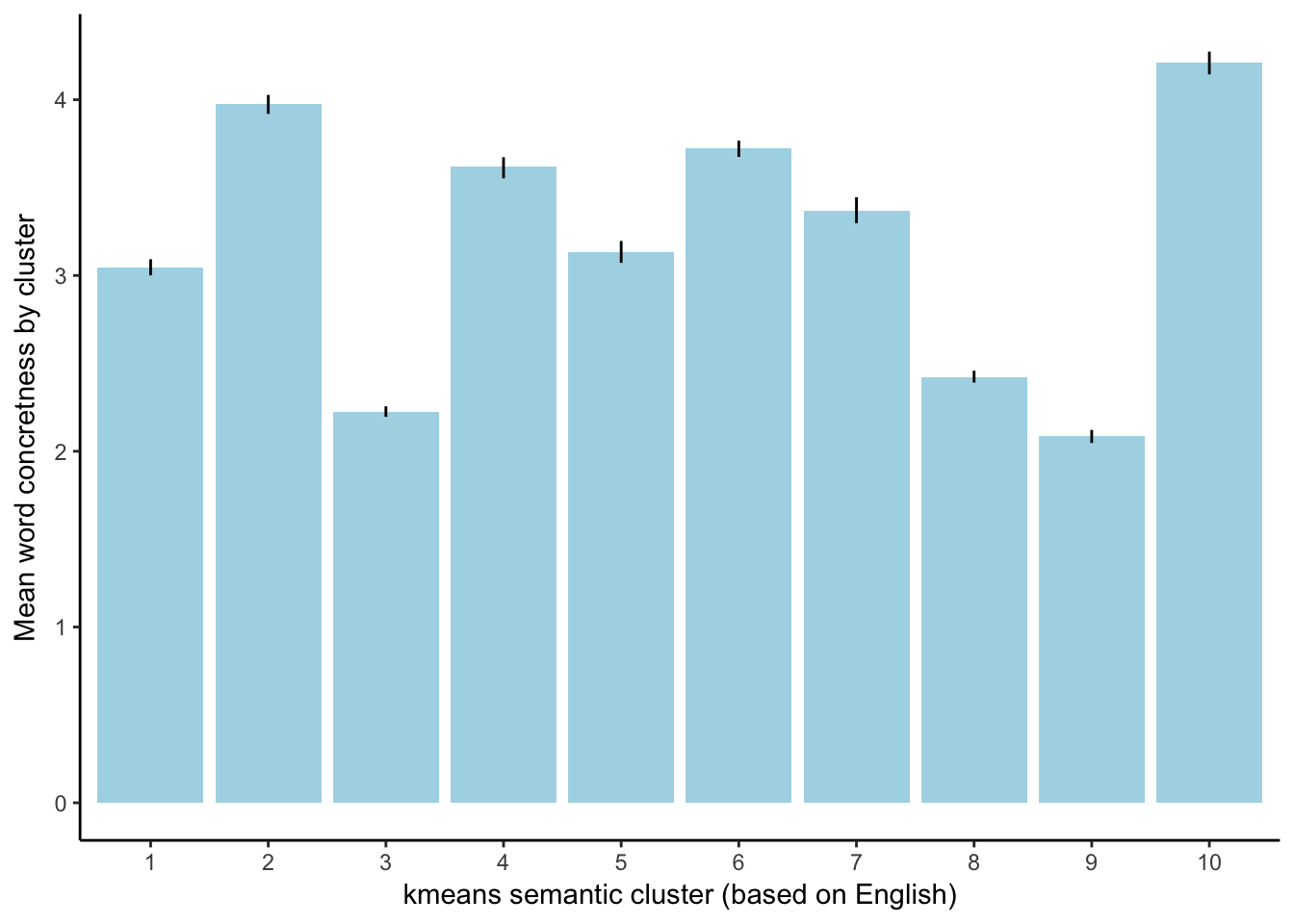## Analysis 1: In vs. across semantic distances

I clustered the 10000 words used in the concreteness analysis into 10 cluster using kmeans. Then for each language, for each cluster, calculated the cosine distance between all within-cluster members and between in and out cluster members. Then, for each language pair, for each cluster, calculated the correlation between in cluster distances and the correlation between out cluster distances. Finally, I averaged across language pairs for each cluster and group, plotted below:

``````semantics_corrs <- read_csv("data/lang_pairwise_semantics_correlations.csv" ,
col_names = c("cluster", "group", "pearsons_r", "lang1", "lang2"))

cluster_corrs <- semantics_corrs %>%
group_by(cluster, group) %>%
multi_boot_standard(col = "pearsons_r")

ggplot(cluster_corrs, aes( x = as.factor(cluster), y = mean, group = group, fill = group)) +
geom_bar(position = "dodge", stat = "identity") +
ylab("mean cross-linguistic \ncorrelation (pearson's r)") +
xlab("kmeans semantic cluster (based on English)")  +
geom_linerange(aes(ymin = ci_lower, ymax = ci_upper),
position=position_dodge(width=0.9)) +
theme_classic()``````## Analysis 2: Semantic centroid distances.

How correlated are the distances between cluster centroids across languages?

Each unit here is a language pair.

``````CENTROID_PATH <- "data/xling_centroid_distance_correlations.csv"

ggplot(centroid_corrs, aes(x = pearsons_r)) +
ggtitle("Language pairwise centroid correlations") +
geom_histogram() +
theme_classic()``````## Analysis 3: Concreteness across English clusters

``````cluster_assignments_raw <- read_csv("data/target_word_cluster_assignments.csv")

cluster_assignments <- cluster_assignments_raw %>%
left_join(concretness %>% select(Word, Conc.M), by = c("word" = "Word"))

cluster_conc_means <- cluster_assignments %>%
group_by(cluster) %>%
multi_boot_standard(col = "Conc.M")

ggplot(cluster_conc_means,
aes(x = as.factor(cluster),
y = mean)) +
geom_bar(position = "dodge", stat = "identity", fill = "lightblue") +
ylab("Mean word concretness by cluster") +
xlab("kmeans semantic cluster (based on English)")  +
geom_linerange(aes(ymin = ci_lower, ymax = ci_upper),
position=position_dodge(width=0.9)) +
theme_classic()``````### Semantics x concreteness

``````conc_assignments <- read_csv("data/target_translations_xling_words.csv") %>%
distinct(word, concreteness_tile)

all_assignments <- full_join(conc_assignments, cluster_assignments_raw)

bin_ns <- all_assignments %>%
group_by(concreteness_tile, cluster) %>%
summarize(n = n())

ggplot(bin_ns, aes(x = concreteness_tile, y = cluster, fill = n)) +
geom_tile() +
xlab("concreteness decile") +
ylab("semantics cluster") +
scale_fill_continuous(low = "white", high = "red") +
scale_x_continuous(breaks=1:10) +
scale_y_continuous(breaks=1:10)  +
theme_classic()``````